•••••••••••••••••••••••••••••••••••••••••••••••••••••••••••••••••••••••••• Home /Exam Details (QP Included) / Main Exam / Optional Subject-Medical Group / Physics / W.B.C.S. Notes On – Physics – Spherical Lenses.
•Posted on June 10th, 2019 in Physics
Tags:

W.B.C.S. Notes On – Physics – Spherical Lenses.

• A transparent material (normally glass) bound by two surfaces, of which one or both surfaces are spherical, is known as “spherical lens.”Continue Reading W.B.C.S. Notes On – Physics – Spherical Lenses.

Convex Lens

• A lens may have two spherical surfaces, bulging outwards (as shown in the image given below), is known as convex lens or a double convex lens.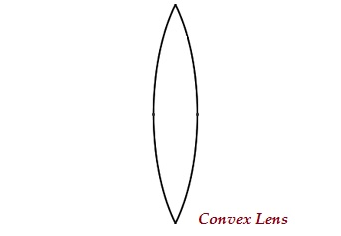• The middle part of this lens is bulged (thicker) and at the both ends, it is narrow.
• Convex lens converges the light rays; therefore, it is also known as converging lens.

Concave Lens

• A lens may have two spherical surfaces, curved inwards (as shown in the image given below), is known as concave lens or a double concave lens.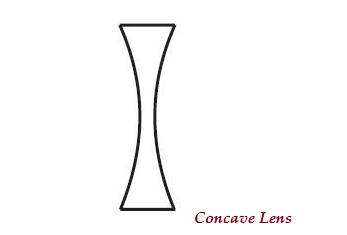• The middle part of this lens is narrow (curved inwards) and the both the edges are thicker.
• Concave lens diverges the light rays; therefore, it is also known as diverging lens.
• A lens, either a concave or a convex, has two spherical surfaces and each of these surfaces forms a part of the sphere. The centers of these spheres are known as centers of curvature, represented by English letter ‘C.’
• As there are two centers of curvature, therefore, represented as ‘C1’ and ‘C2.’
• An imaginary straight line, passing through both the centers of curvature of a lens, is known as principal axis.
• Optical center is the central point of a lens. It is represented by ‘O.’
• An aperture is the actual diameter of the circular outline of a spherical lens.
• Principal focus of lens is represented by ‘F.’
• A lens has usually two foci represented as F1 and F2.
• Focal length is the distance between the principal focus and the optical center of a lens. It is represented by ‘f.’
• The following table illustrates, the nature and position of images formed by a convex lens −
Position of Object Position of Image Size of Image Nature of Image Image
At infinity At the focus F2 Highly diminished, pointsized Real and inverted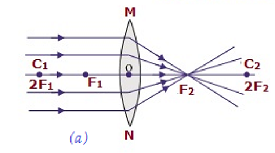Beyond 2F1 B/w F2 and 2F2 Diminished Real and inverted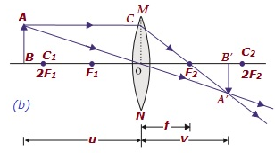At 2F1 At 2F2 Same size Real and inverted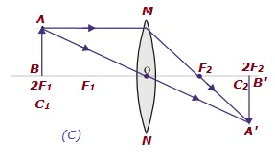B/w F1 & 2F1 Beyond 2F2 Enlarged Real and inverted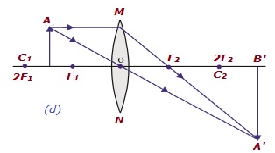At focus F1 At infinity Infinitely large or highly enlarged Real & inverte d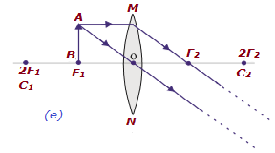B/w focus F1& optical center O On the same side of the lens as the object Enlarged Virtual and erect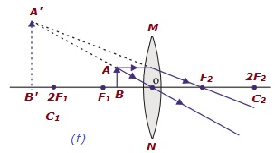• The following table illustrates, the nature and position of images formed by a concave lens −
Position of Object Position of Image Relative Size of Image Nature of Image Image
At infinity At the focus F1 Highly diminishe d, pointsized Virtual and erect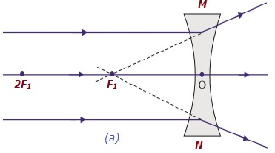B/w infinity & optical center O of the lens B/w F1 & optical center O Diminishe d Virtual and erect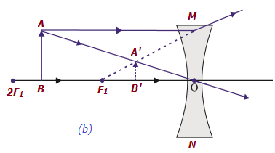Lens Formula

• The formula is expressed as −
1v1u=1f1v−1u=1f
• Lens formula expresses the relationships among the object-distance (i.e. u), image-distance (i.e. v), and focal length (i.e. f) of a lens.